Home
Hostname: page-component-544b6db54f-kbvt8 Total loading time: 0.216 Render date: 2021-10-16T00:53:18.921Z Has data issue: true Feature Flags: { "shouldUseShareProductTool": true, "shouldUseHypothesis": true, "isUnsiloEnabled": true, "metricsAbstractViews": false, "figures": true, "newCiteModal": false, "newCitedByModal": true, "newEcommerce": true, "newUsageEvents": true }Compositio Mathematica

# On fields of rationality for automorphic representations

Published online by Cambridge University Press:  11 September 2014

## Abstract

HTML view is not available for this content. However, as you have access to this content, a full PDF is available via the ‘Save PDF’ action button.

This paper proves two results on the field of rationality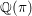$\mathbb{Q}({\it\pi})$ for an automorphic representation${\it\pi}$, which is the subfield of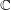$\mathbb{C}$ fixed under the subgroup of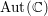$\text{Aut}(\mathbb{C})$ stabilizing the isomorphism class of the finite part of${\it\pi}$. For general linear groups and classical groups, our first main result is the finiteness of the set of discrete automorphic representations${\it\pi}$ such that${\it\pi}$ is unramified away from a fixed finite set of places,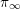${\it\pi}_{\infty }$ has a fixed infinitesimal character, and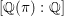$[\mathbb{Q}({\it\pi}):\mathbb{Q}]$ is bounded. The second main result is that for classical groups,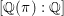$[\mathbb{Q}({\it\pi}):\mathbb{Q}]$ grows to infinity in a family of automorphic representations in level aspect whose infinite components are discrete series in a fixed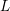$L$-packet under mild conditions.

## MSC classification

Type
Research Article
Information
Compositio Mathematica , December 2014 , pp. 2003 - 2053

## References

Anderson, G., Blasius, D., Coleman, R. and Zettler, G., On representations of the Weil group with bounded conductor, Forum Math. 6 (1994), 537545.CrossRefGoogle Scholar
Arthur, J., Orthogonal and symplectic groups, in The endoscopic classification of representations, American Mathematical Society Colloquium Publications, vol. 61 (American Mathematical Society, Providence, RI, 2013).CrossRefGoogle Scholar
Barnet-Lamb, T., Gee, T., Geraghty, D. and Taylor, R., Local–global compatibility for l = p. II, Ann. Sci. Éc. Norm. Supér. (4) 47 (2014), 165179.CrossRefGoogle Scholar
Bergeron, N., Millson, J. and Moeglin, C., Hodge type theorems for arithmetic manifolds associated to orthogonal groups, Ann. of Math. (2), submitted. Preprint (2011),arXiv:1110.3049.Google Scholar
Blasius, D., Harris, M. and Ramakrishnan, D., Coherent cohomology, limits of discrete series, and Galois conjugation, Duke Math. J. 73 (1994), 647685.CrossRefGoogle Scholar
Borel, A., Automorphic L-functions, in Automorphic forms, representations and L-functions (Oregon State University, Corvallis, OR, 1977, Part 2), Proceedings of Symposia in Pure Mathematics, vol. XXXIII (American Mathematical Society, Providence, RI, 1979), 2761.Google Scholar
Borel, A., Automorphic forms on reductive groups, in Automorphic forms and applications, IAS/Park City Mathematics Series, vol. 12 (American Mathematical Society, Providence, RI, 2007), 739.Google Scholar
Buzzard, K. and Gee, T., The conjectural connections between automorphic representations and Galois representations, in Proc. LMS Durham Symp. (2011), to appear. Preprint (2010),arXiv:1009.0785.Google Scholar
Calegari, F. and Emerton, M., The Hecke algebra T khas large index, Math. Res. Lett. 11 (2004), 125137.CrossRefGoogle Scholar
Caraiani, A., Monodromy and local–global compatibility for$l=p$, Preprint (2012),arXiv:1202:4683.Google Scholar
Caraiani, A., Local–global compatibility and the action of monodromy on nearby cycles, Duke Math. J. 161 (2012), 23112413.CrossRefGoogle Scholar
Chenevier, G. and Harris, M., Construction of automorphic Galois representations, II, Cambridge Math. J. 1 (2013), 5373.CrossRefGoogle Scholar
Clozel, L., Motifs et formes automorphes: applications du principe de fonctorialité, in Automorphic forms, Shimura varieties, and L-functions, Vol. I (Ann Arbor, MI, 1988, Perspectives in Mathematics, vol. 10 (Academic Press, Boston, MA, 1990), 77159.Google Scholar
Clozel, L., Purity reigns supreme, Int. Math. Res. Not. IMRN (2013), 328346.CrossRefGoogle Scholar
Deitmar, A. and Hoffman, W., Spectral estimates for towers of noncompact quotients, Canad. J. Math. 51 (1999), 266293.CrossRefGoogle Scholar
Deligne, P., Théorie de Hodge. I, in Actes du Congrès International des Mathématiciens (Nice, 1970), Tome 1 (Gauthier-Villars, Paris, 1971), 425430.Google Scholar
Duke, W. and Kowalski, E., A problem of Linnik for elliptic curves and mean-value estimates for automorphic representations, Invent. Math. 139 (2000), 139; with an appendix by Dinakar Ramakrishnan.CrossRefGoogle Scholar
Elkies, N. D., Howe, E. W. and Ritzenthaler, C., Genus bounds for curves with fixed Frobenius eigenvalues, Proc. Amer. Math. Soc. 142 (2014), 7184.CrossRefGoogle Scholar
Ellenberg, J. S. and Venkatesh, A., Reflection principles and bounds for class group torsion, Int. Math. Res. Not. IMRN 2007 (2007), doi:10.1093/imrn/rnm002.Google Scholar
Faltings, G., Finiteness theorems for abelian varieties over number fields, in Arithmetic geometry (Storrs, CT, 1984) (Springer, New York, 1986), 927; translated from the German original [Invent. Math. 73 (1983, no. 3, 349–366; Invent. Math. 75 (1984), no. 2, 381; MR 85g:11026ab] by Edward Shipz.CrossRefGoogle Scholar
Flath, D., Decomposition of representations into tensor products, in Proceedings of Symposia in Pure Mathematics, vol. 33(1) (American Mathematical Society, Providence, RI, 1979), 179183.Google Scholar
Fontaine, J.-M. and Mazur, B., Geometric Galois representations, in Elliptic curves, modular forms, & Fermat’s last theorem (Hong Kong, 1993), Series in Number Theory, vol. I (International Press, Cambridge, MA, 1995), 4178.Google Scholar
Franke, J. and Schwermer, J., A decomposition of spaces of automorphic forms, and the Eisenstein cohomology of arithmetic groups, Math. Ann. 311 (1998), 765790.CrossRefGoogle Scholar
Gamburd, A., Jakobson, D. and Sarnak, P., Spectra of elements in the group ring of SU(2), J. Eur. Math. Soc. (JEMS) 1 (1999), 5185.CrossRefGoogle Scholar
Gross, B. H., Algebraic modular forms, Israel J. Math. 113 (1999), 6193.CrossRefGoogle Scholar
Gross, B. H. and Rohrlich, D. E., Some results on the Mordell–Weil group of the Jacobian of the Fermat curve, Invent. Math. 44 (1978), 201224.CrossRefGoogle Scholar
Harish-Chandra, Automorphic forms on semisimple Lie groups. Notes by J. G. M. Mars, Lecture Notes in Mathematics, vol. 62(Springer, Berlin, 1968).Google Scholar
Harris, M. and Taylor, R., The geometry and cohomology of some simple Shimura varieties, Annals of Mathematics Studies, vol. 151 (Princeton University Press, Princeton, NJ, 2001); with an appendix by Vladimir G. Berkovich.Google Scholar
Helfgott, H. A. and Venkatesh, A., Integral points on elliptic curves and 3-torsion in class groups, J. Amer. Math. Soc. 19 (2006), 527550; (electronic).CrossRefGoogle Scholar
Henniart, G., Une preuve simple des conjectures de Langlands pour GL(n) sur un corpsp-adiques, Invent. Math. 139 (2000), 439455.CrossRefGoogle Scholar
Hida, H., Hecke fields of analytic families of modular forms, J. Amer. Math. Soc. 24 (2011), 5180.CrossRefGoogle Scholar
Hida, H., A finiteness property of abelian varieties with potentially ordinary good reduction, J. Amer. Math. Soc. 25 (2012), 813826.CrossRefGoogle Scholar
Khare, C., Conjectures on finiteness of mod p Galois representations, J. Ramanujan Math. Soc. 15 (2000), 2342.Google Scholar
Kim, H. H. and Krishnamurthy, M., Stable base change lift from unitary groups to GLn, IMRP Int. Math. Res. Pap. 1 (2005), 152.Google Scholar
Kottwitz, R. E. and Shelstad, D., Foundations of twisted endoscopy, Astérisque (1999), vi+190.Google Scholar
Labesse, J.-P., Changement de base CM et séries discrètes, in On the stabilization of the trace formula, The Stable Trace Formula, Shimura Varieties, and Arithmetic Applications, vol. 1 (International Press, Somerville, MA, 2011), 429470.Google Scholar
Langlands, R., The classification of representations of real reductive groups, Mathematics Surveys and Monographs, vol. 31 (American Mathematical Society, Providence, RI, 1988).Google Scholar
Langlands, R. and Shelstad, D., On the definition of transfer factors, Math. Ann. 278 (1987), 219271.CrossRefGoogle Scholar
Madan, M. L. and Madden, D. J., Note on class groups of algebraic function fields, J. Reine Angew. Math. 295 (1977), 5760.Google Scholar
Milne., J. S., Abelian varieties, in Arithmetic geometry (Storrs, CT, 1984) (Springer, New York, 1986), 103150.CrossRefGoogle Scholar
Moeglin, C., Classification et changement de base pour les séries discrètes des groupes unitaires padiques, Pacific J. Math. 233 (2007), 159204.CrossRefGoogle Scholar
Mok, C. P., Endoscopic classification of representations of quasi-split unitary groups, Mem. Amer. Math. Soc., to appear. Preprint (2012), arXiv:1206.0882.Google Scholar
Ngô., B. C., Le lemme fondamental pour les algèbres de Lie, Publ. Math. Inst. Hautes Études Sci. (2010), 1169.CrossRefGoogle Scholar
Patrikis, S., Variations on a theorem of Tate, Preprint (2012), arXiv:1207.6724v1 [math.NT].Google Scholar
Rohlfs, J. and Speh, B., On limit multiplicities of representations with cohomology in the cuspidal spectrum, Duke Math. 55 (1987), 199211.CrossRefGoogle Scholar
Royer, E., Facteurs Q-simples de J 0(N) de grande dimension et de grand rang, Bull. Soc. Math. France 128 (2000), 219248.CrossRefGoogle Scholar
Saito, T., Weight spectral sequences and independence of l, J. Inst. Math. Jussieu 2 (2003), 583634.CrossRefGoogle Scholar
Sarnak, P., Maass cusp forms with integer coefficients, in A panorama of number theory or the view from Baker’s garden (Zürich, 1999) (Cambridge University Press, Cambridge, 2002), 121127.Google Scholar
Sauvageot, F., Principe de densité pour les groupes réductifs, Compositio Math. 108 (1997), 151184.CrossRefGoogle Scholar
Scholze, P., Perfectoid spaces, Publ. Math. Inst. Hautes Études Sci. 116 (2012), 245313.CrossRefGoogle Scholar
Schwermer, J., Geometric cycles, arithmetic groups and their cohomology, Bull. Amer. Math. Soc. (N.S.) 47 (2010), 187279.CrossRefGoogle Scholar
Serre, J.-P., Linear representations of finite groups, Graduate Texts in Mathematics, vol. 42 (Springer, New York, 1977), translated from the second French edition by Leonard L. Scott.CrossRefGoogle Scholar
Serre, J.-P., Local fields, Graduate Texts in Mathematics, vol. 67 (Springer, New York, 1979), translated from the French by Marvin Jay Greenberg.CrossRefGoogle Scholar
Serre, J.-P., Répartition asymptotique des valeurs propres de l’opérateur de Hecke T p, J. Amer. Math. Soc. 10 (1997), 75102.CrossRefGoogle Scholar
Serre, J.-P., Bounds for the orders of the finite subgroups of G (k), in Group representation theory (EPFL Press, Lausanne, 2007), 405450.Google Scholar
Shin, S. W., Galois representations arising from some compact Shimura varieties, Ann. of Math. (2) 173 (2011), 16451741.CrossRefGoogle Scholar
Shin, S. W., Automorphic Plancherel density theorem, Israel J. Math. 192 (2012), 83120.CrossRefGoogle Scholar
Shin, S.-W. and Templier, N., Sato–Tate theorem for families and low-lying zeros of automorphic L-functions, submitted, 129pp, with Appendix A by R. Kottwitz and Appendix B by R. Cluckers, J. Gordon and I. Halupczok. Preprint (2012), arXiv:1208.1945.Google Scholar
Taylor, R., Galois representations, Ann. Fac. Sci. Toulouse 13 (2004), 73119.CrossRefGoogle Scholar
Taylor, R. and Yoshida, T., Compatibility of local and global Langlands correspondences, J. Amer. Math. Soc. 20 (2007), 467493.CrossRefGoogle Scholar
Vaaler, J. D., Some extremal functions in Fourier analysis, Bull. Amer. Math. Soc. (N.S.) 12 (1985), 183216.CrossRefGoogle Scholar
Vogan, D. A. Jr, The local Langlands conjecture, in Representation theory of groups and algebras, Contemporary Mathematics, vol. 145 (American Mathematical Society, Providence, RI, 1993), 305379.CrossRefGoogle Scholar
Waldspurger, J.-L., Quelques propriétés arithmétiques de certaines formes automorphes sur GL(2), Compositio Math. 54 (1985), 121171.Google Scholar
Waldspurger, J.-L., Le lemme fondamental implique le transfert, Compositio Math. 105 (1997), 153236.CrossRefGoogle Scholar
Waldspurger, J.-L., La formule de Plancherel pour les groupes p-adiques d’après Harish-Chandra, J. Inst. Math. Jussieu 2 (2003), 235333.CrossRefGoogle Scholar
Waldspurger, J.-L., Endoscopie et changement de caractéristique, J. Inst. Math. Jussieu 5 (2006), 423525.CrossRefGoogle Scholar
Waldspurger, J.-L., L’endoscopie tordue n’est pas si tordue, Mem. Amer. Math. Soc. 194 (2008), x+261.Google Scholar
Waldspurger, J.-L., Les facteurs de transfert pour les groupes classiques: un formulaire, Manuscripta Math. 133 (2010), 4182.CrossRefGoogle Scholar
Yamada, T., A remark on Schur indices of p-groups, Proc. Amer. Math. Soc. 76 (1979), 45.Google Scholar
Yu, J.-K., Bruhat–Tits theory and buildings, in Ottawa lectures on admissible representations of reductive p-adic groups, Fields Institute Monographs, vol. 26 (American Mathematical Society, Providence, RI, 2009), 5377.Google ScholarYou have Access
8
Cited by

# Send article to Kindle

Note you can select to send to either the @free.kindle.com or @kindle.com variations. ‘@free.kindle.com’ emails are free but can only be sent to your device when it is connected to wi-fi. ‘@kindle.com’ emails can be delivered even when you are not connected to wi-fi, but note that service fees apply.

Find out more about the Kindle Personal Document Service.

On fields of rationality for automorphic representations
Available formats
×

# Send article to Dropbox

To send this article to your Dropbox account, please select one or more formats and confirm that you agree to abide by our usage policies. If this is the first time you use this feature, you will be asked to authorise Cambridge Core to connect with your <service> account. Find out more about sending content to Dropbox.

On fields of rationality for automorphic representations
Available formats
×

# Send article to Google Drive

To send this article to your Google Drive account, please select one or more formats and confirm that you agree to abide by our usage policies. If this is the first time you use this feature, you will be asked to authorise Cambridge Core to connect with your <service> account. Find out more about sending content to Google Drive.

On fields of rationality for automorphic representations
Available formats
×
×